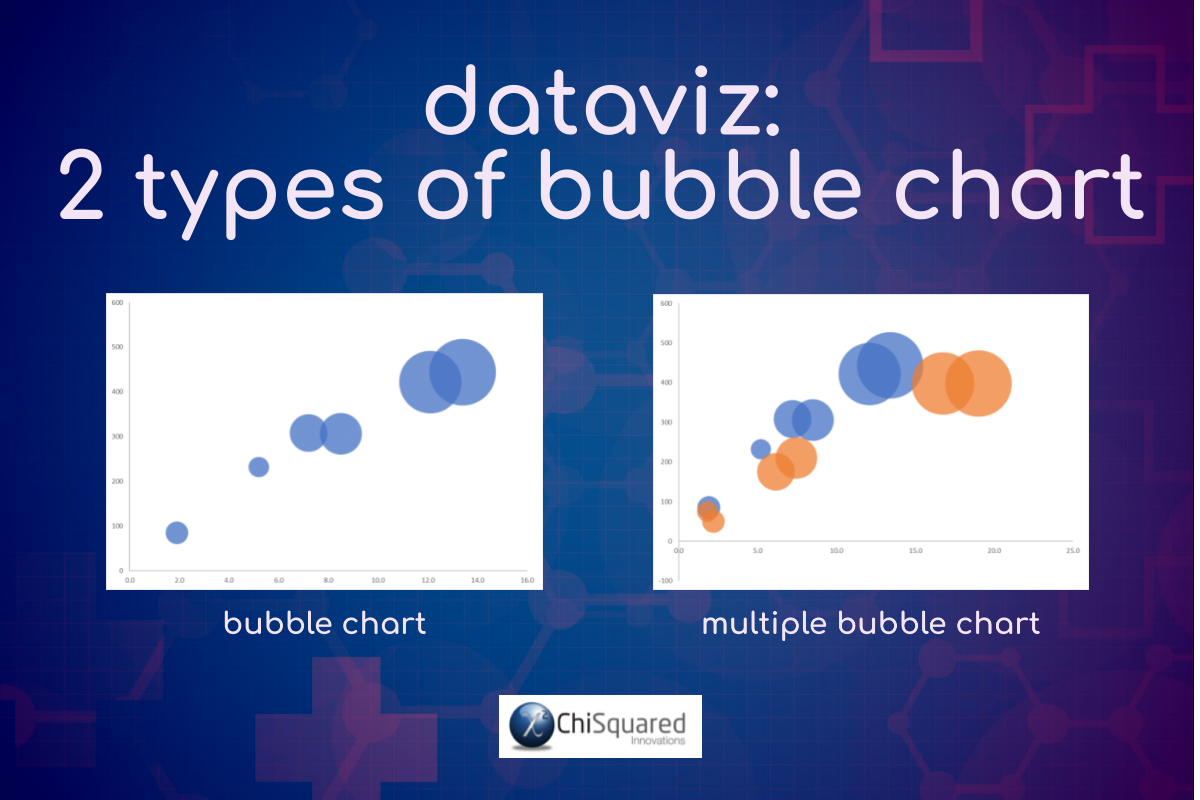July 21

# DataViz – How to Choose the Right Chart for Your Data

Blog, Discover Visualisation

Data visualisation is sexy – probably the sexiest part of statistics, Data Science and business intelligence.

And it can make or break your study!

Few people that have to create charts as part of their study know and understand how to create charts for maximum effectiveness, and as a result struggle to engage their audience.

And that’s a shame, because a well-crafted chart has the potential to change the world!

Charts really are that important!

Disclosure: we may earn an affiliate commission for purchases you make when using the links to products on this page. As an Amazon Affiliate we earn from qualifying purchases.

In this guide on how to choose the right chart for your data, I’ll explain about the different types of graphs you’ll use.

Then I’ll show you which data goes on which axis.

I’ll show you the different types of charts to use, and how to choose which ones to use.

We’ll move on to how to style your charts and review them with a critical eye to decide what should go on your graph, and – more importantly – what you should take off.

Finally, I’ll introduce you to DataViz – The Big Picture, a FREE Ultra-High-Definition flowchart that will guarantee that you get the right chart first time, every time!

(psstt - don't tell anyone, but you can get a FREE copy right here:)

FREE DataViz Flowchart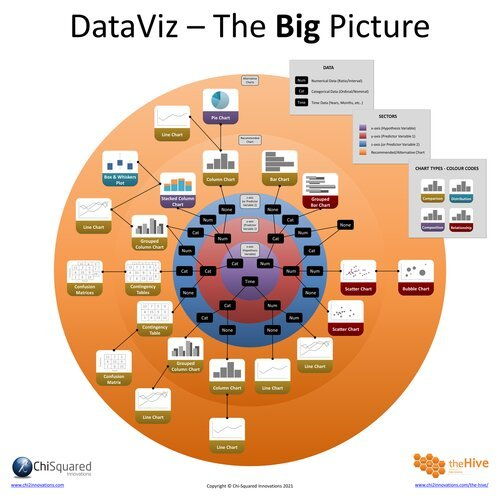Master the Fundamentals of Data Visualisation

Ultra-Hi-Definition PDF

By the time you’ve read this dataviz guide, you’ll know more about plotting charts than pretty much everyone around you!

This post is part of a series of articles about the most used types of graphs in statistics for presenting data.

You can use the following jump-station to choose the content you're looking for (and there will be another jump-station at the bottom of this post):

DataViz Jump-Station

This post is part of a series on the most used graphs in statistics.

For more detail, choose from the options below:

DataViz:

How to Choose The Right Chart for Your Data

## How Many Variables Can Be on a Graph?

One of the first problems that most people encounter when they’re plotting graph variables is deciding how many variables to add to their graph – particularly if they’re in the exploratory phase of their analyses rather than at the story-telling stage.

Before you can decide how many graph variables to include, it’s useful to understand their data types, and this will have a huge influence on how many graph variables you can include – and on which types of graphs you can use.

There are just 3 types of data graphs in statistics:

• Numerical Data Graphs
• Categorical Data Graphs
• Time Data Graphs

### Numerical Data Graphs

Numerical data graphs (aka quantitative data graphs or graphs for continuous data) are the graph types on which we are plotting only numerical data (on both the x- and y-axes).

Height (e.g. 1.6m) and Weight (e.g. 263kg) are examples of numerical data (aka continuous data or quantitative data).

So which graphs are used to plot continuous data? Well, we’re plotting numerical data on both axes, but it is important to note that the feature of interest is always plotted on the y-axis (more on that in the next section).

#### Numerical Data Graphs - Example

Examples of numerical data graphs include:

• Scatter Charts
• Bubble Charts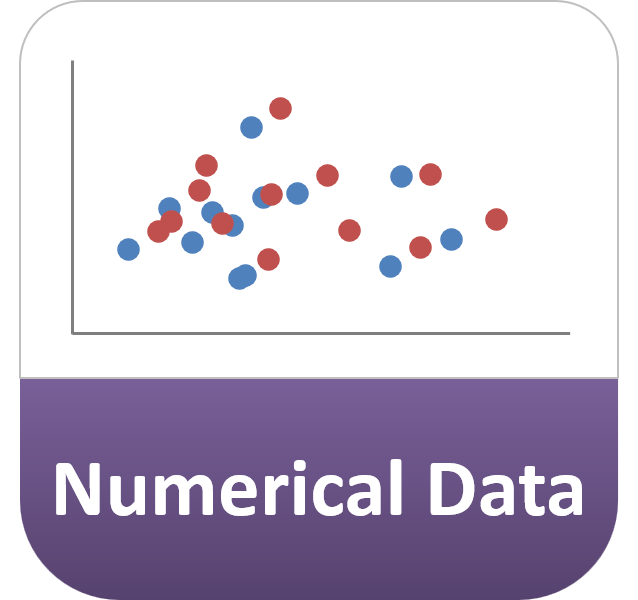### Categorical Data Graphs

Categorical data are data arranged in categories, such as Size [Small, Medium, Large], and graphs using categorical data are called categorical data graphs.

#### Categorical Data Graphs - Example

Examples of categorical data graphs where the x-axis is arranged in categories (and the numerical feature of interest variable is on the y-axis) include:

• Column Charts
• Stacked Column Charts
• Grouped Column Charts

Examples of categorical data graphs where the categorical feature of interest is plotted on the y-axis and the numerical variable is on the x-axis include:

• Bar Charts
• Stacked Bar Charts
• Grouped Bar Charts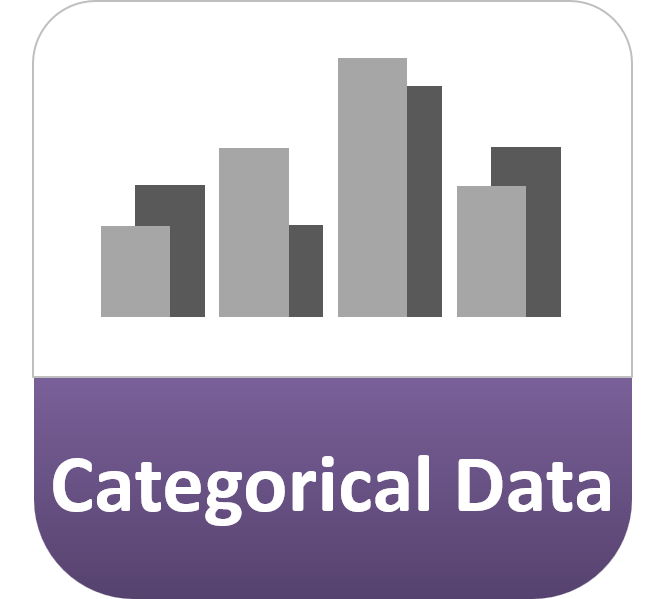### Time Data Graphs

When Time is the feature of interest, such as how long it takes to run the 100 metres, then you would usually consider Time as a numerical variable and plot it on the y-axis. The type of graph you use depends on whether your x-axis is a numerical variable or categorical variable (as discussed above).

On the other hand, when you want to see how the feature of interest varies over time, you plot your variable against time on a Time Data Graph. In this case, Time is always plotted on the x-axis, with the variable(s) of interest plotted on the y-axis.

#### Time Data Graphs - Example

Examples of time data graphs include:

• Line Charts
• Column Charts
• Bar Charts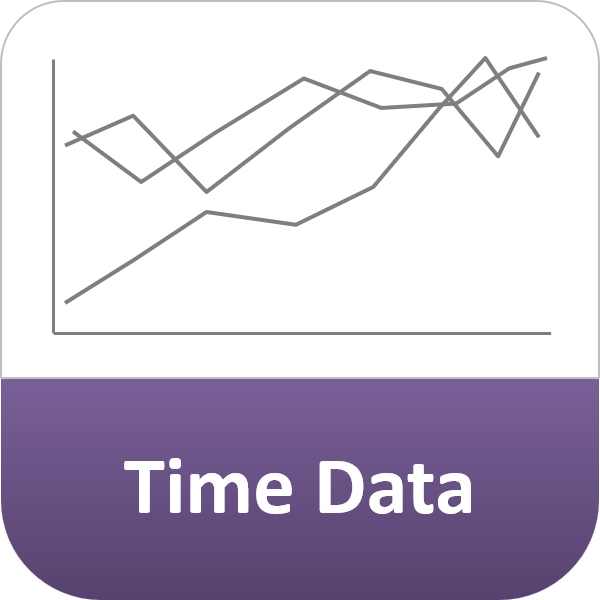### 3 Data Type Graphs - Summary

So, as a reminder, here are the 3 different data type graphs again: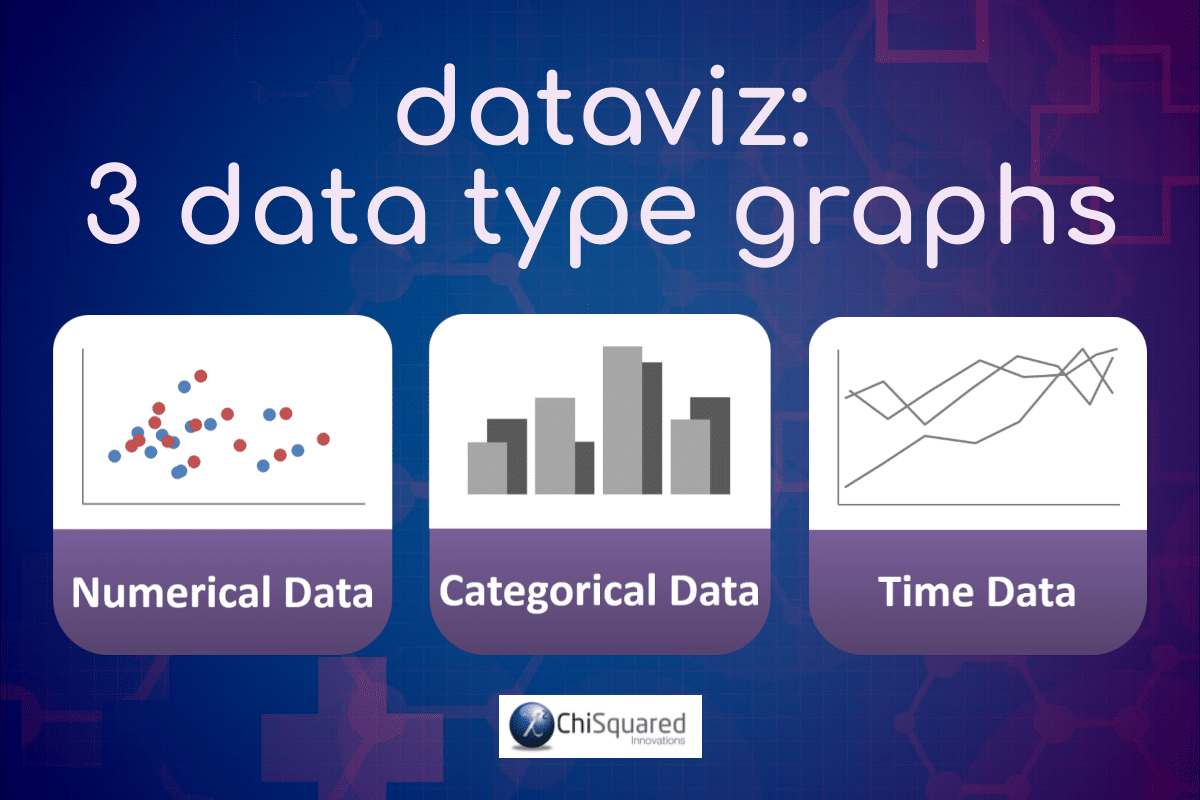### So How Many Variables Can Be on a Graph?

The unfortunate answer to this question is that there is no limit to the number of variables you can add to your graphs. Whether you’re using a Bar Chart, a Column Chart, a Scatter Chart or a Line Chart, you can plot as many graph variables as you wish!

But why would you want to?

The more graph variables you plot, the harder it is to see patterns and trends. At an early investigation stage, it might be useful to plot lots of variables on a single chart to see if you can spot an outlier, but mostly you’ll just end up with a lot of confusing mush!

Try to limit the number of variables on your graphs to the smallest number needed to tell the story of your data.

And don’t forget, if a picture paints a thousand words, then two pictures tell twice the story. Plotting more graphs with fine detail is better than plotting few graphs full of confusion!

DataViz - How to Choose the Right Chart for Your Data @chi2innovations #datavisualization #charts

## How Do You Know Which Variable to Put on Which Axis?

The variable that contains the feature of interest is known as the Response Variable (aka dependant variable, y-variable, outcome variable or hypothesis variable).

The Response Variable is always plotted on the y-axis.

On any graph, there will only ever be one Response Variable, but there may be multiple Predictor Variables (aka independent variable, x-variable, input variable or explanatory variable).

The Predictor variable is always plotted on the x-axis.

The bottom line here is to decide which variable you’re interested in studying.

If you’re studying how the Weight of schoolkids (of the same age) is affected by their Height, then Weight is the variable of interest and goes on the y-axis. Height then goes on the x-axis and you would plot them using a Scatter Chart, or on a Column Chart if the Heights are categorised.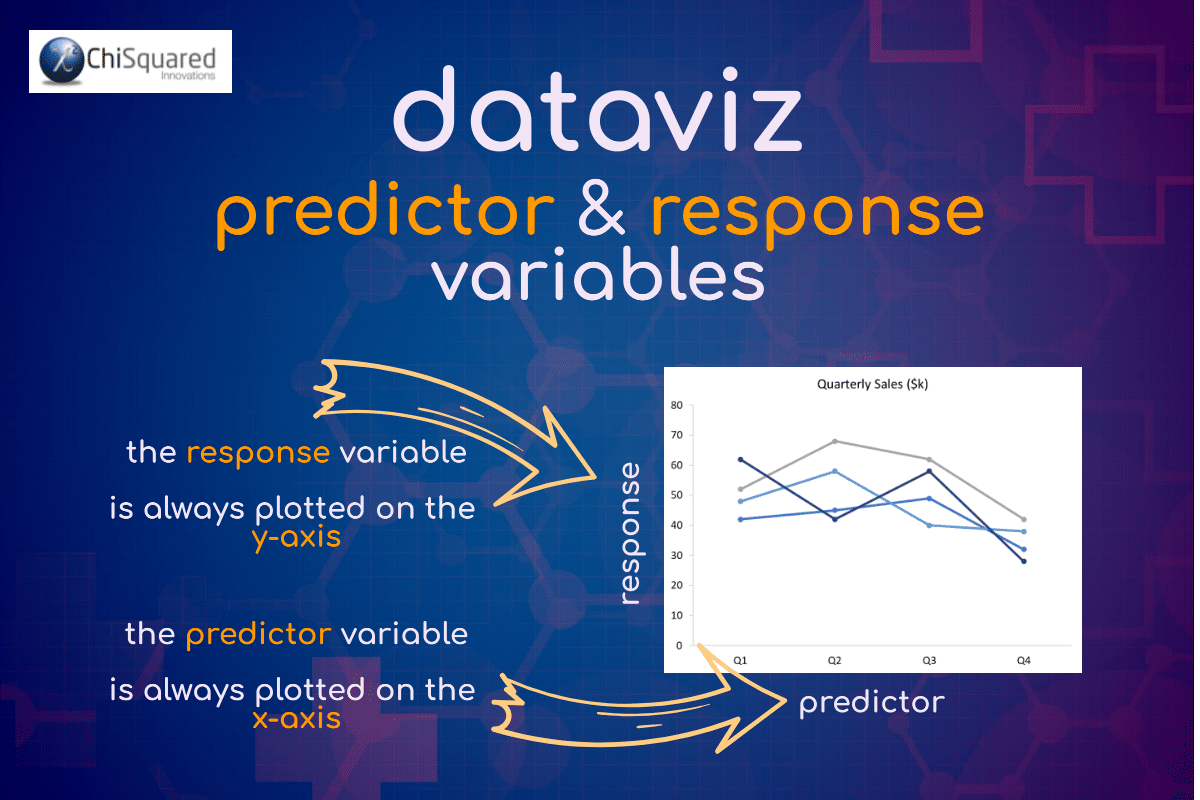## How Do You Decide Which Type of Chart to Use?

Asking the right questions will form the basis for choosing the right data visualisation chart types for your data.

There are 4 questions to ask when deciding which type of chart to use:

1. 1
Do you want to compare variables?
2. 2
Do you want to show what a variable is composed of?
3. 3
Do you want to show the distribution of your data?
4. 4
Are you interested in analysing trends or relationships in your data?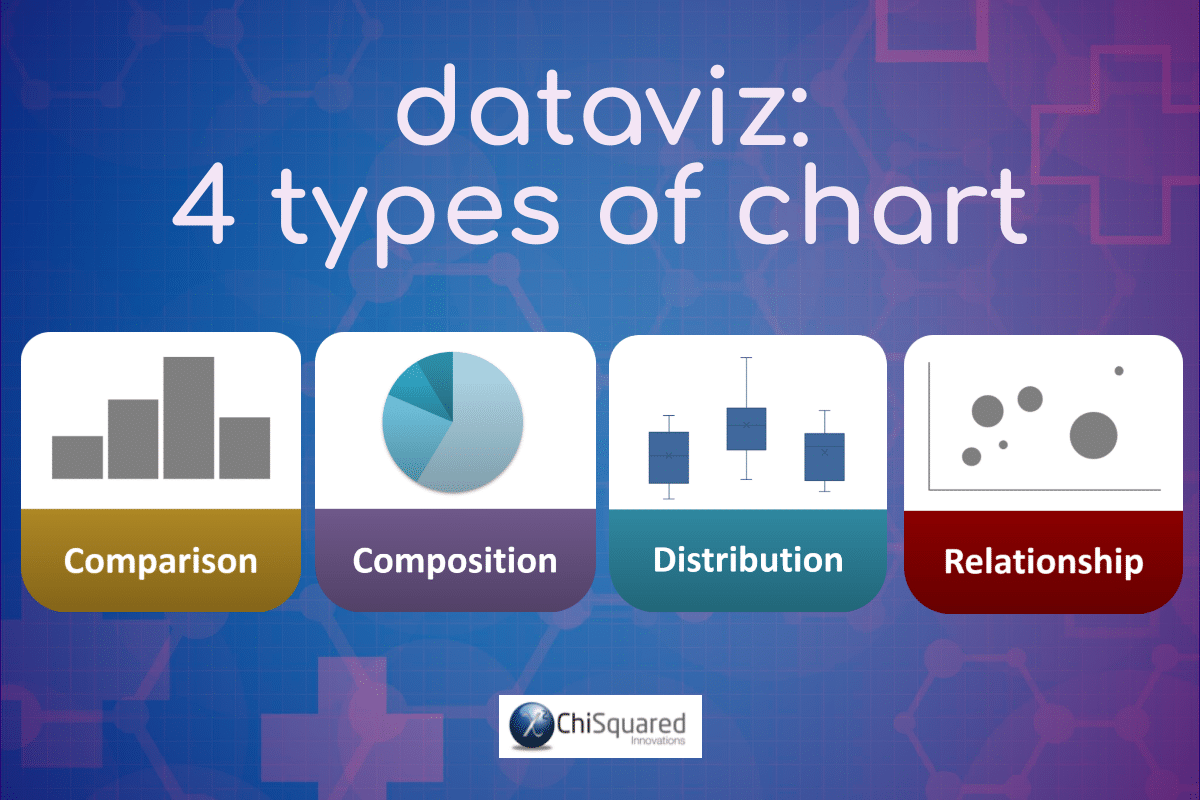Let’s delve a little deeper…

### Do You Want to Compare Variables?

Charts are great for comparing variables or values within variables, and are ideal for showing highs and lows, outliers, before and after, and for showing how variables differ from each other. Typically, the types of charts used to compare data are:

• Line Charts
• Column Charts

### Do You Want to Show What a Variable is Composed of?

If your aim is to show how individual parts make up the whole, then you need to use the right types of data visualisation to achieve this, charts such as:

• Pie Chart
• Stacked Column Chart

### Do You Want to Show the Distribution of Your Data?

Distribution charts help you to understand how your data are distributed, outliers, and where the centre of the distribution is. Mostly, distribution charts are used to assess individual variables, but they can also be used to compare variables too. One such data visualization chart type is:

• Box and Whiskers Plot

### Are You Interested in Analysing Trends or Relationships in Your Data?

If you are interested to know how a variable changed over time or as a result of its interaction with another, then you are investigating their relationship. These types of graphs in statistics are the most common and you will come across them time and again. Appropriate charts include:

• Scatter Chart
• Line Chart
• Bubble Chart
##### VIDEO COURSE

Statistics:

The Big Picture

Free to try - no need to buy or register!

## 8 Types of Graphs in Statistics for Presenting Data

Although there are lots of data visualisation chart types, essentially there are only 8 main types of graphs in statistics for presenting data. If you master when and how to use these, you will have most of dataviz sewn up!

The 8 types of graphs in statistics for presenting data are:

1. 1
Column Chart or Bar Chart
2. 2
Line Chart
3. 3
Scatter Plot
4. 4
Bubble Chart
5. 5
Pie Chart
6. 6
Box and Whiskers Plot
7. 7
Contingency Table
8. 8
Confusion Matrix

Let’s jump in and take a look at each of them.

### Column Chart vs Bar Chart

Firstly, we need to ask what is the difference between Column Chart vs Bar Chart. Yes, they are different beasts!

A Column Chart has vertical columns, whereas a Bar Chart has horizontal columns.

Simple, really!

Ah, yes, but when do you use each one?

Remember earlier when I said that your variable of interest goes on the y-axis? Well, that helps you answer this question.

You use Column and Bar Charts when one of your variables is categorical and the other is numerical.

#### Column Chart vs Bar Chart

When should you use a Column Chart? When your variable of interest is numerical it goes on the y-axis, the categorical variable goes on the x-axis, and you should use a Column Chart.

When should you use a Bar Chart? When your variables are the other way round and your variable of interest is categorical, your numerical variable goes on the x-axis and categorical variable on the y-axis – you should use a Bar Chart.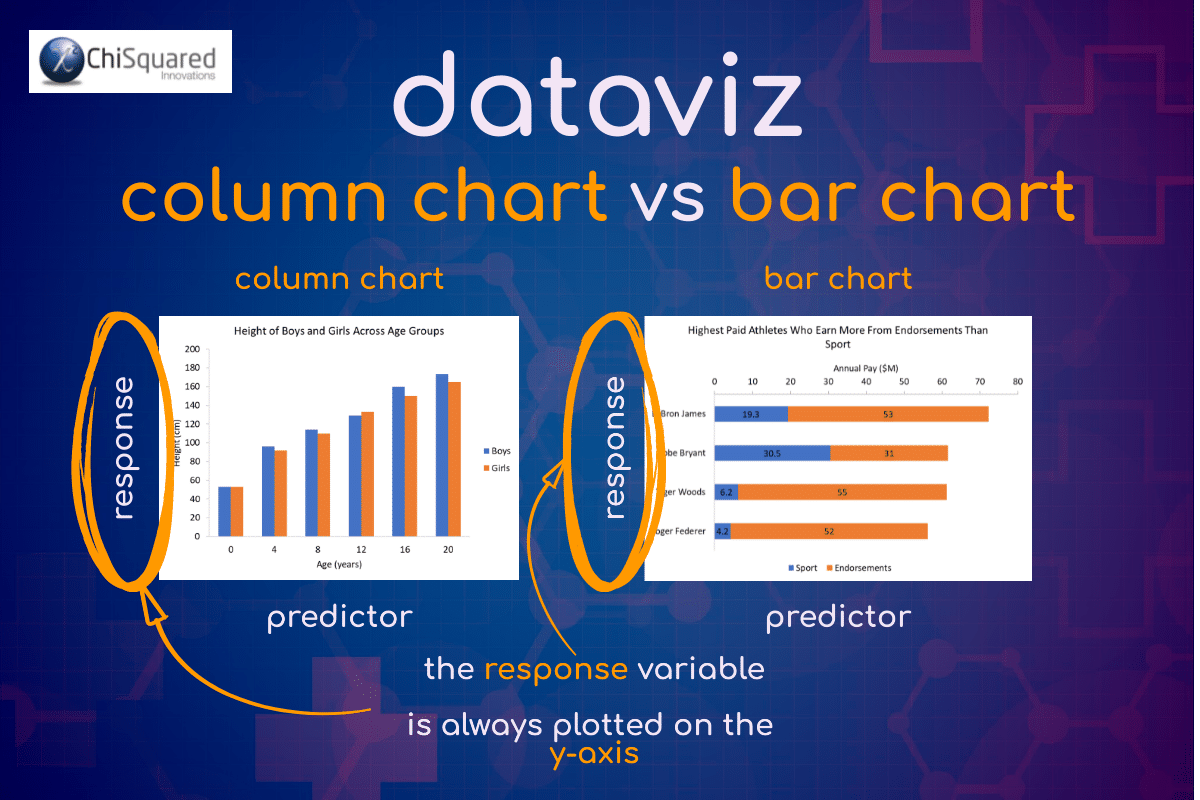#### What does a Column Chart show?

A Column Chart summarises categorical data using vertical bars to represent the quantities of data for each category or it can show data changes over time by displaying a comparison among subjects.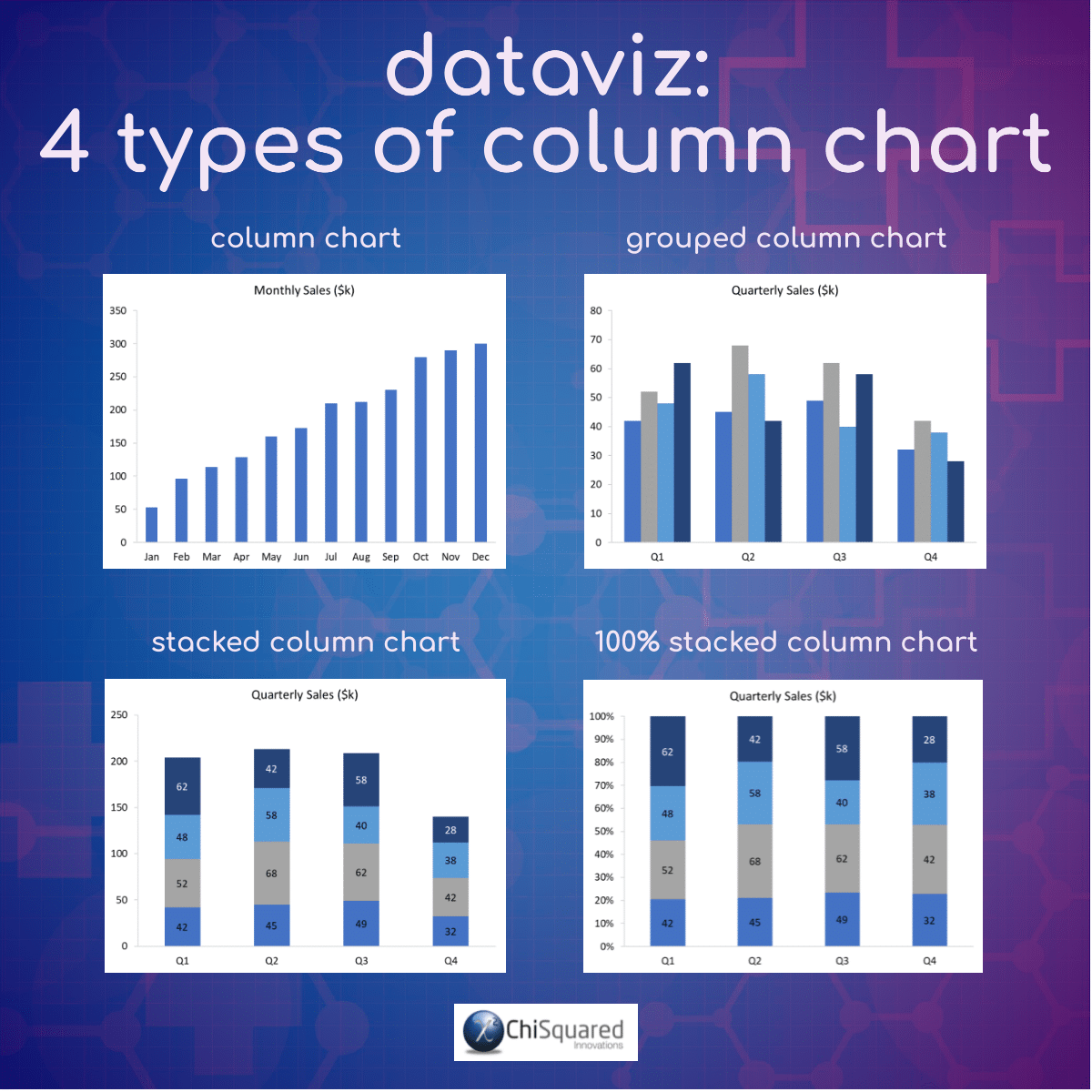If you would like to learn more about Column Charts, you can find a much more in-depth article via our DataViz Jump-Station (at the top and bottom of this post) or by clicking below (opens in a new tab):

## Column Charts – How to Master the Most Used Chart in DataViz

#### What does a Bar Chart show?

A Bar Chart summarises categorical data using horizontal bars to represent the quantities of data for each category. Bar Charts are not usually used to show data changes over time.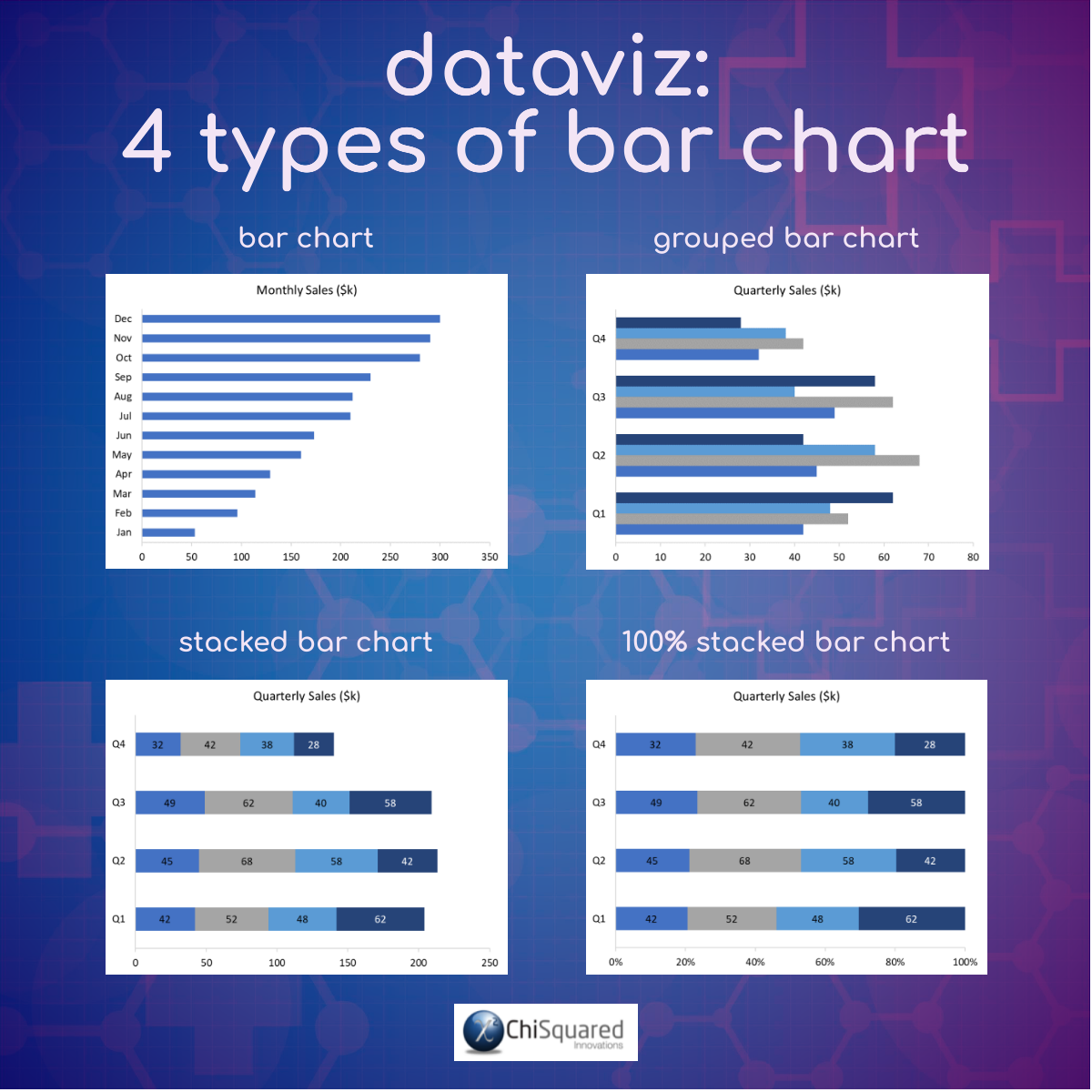If you would like to learn more about Bar Charts, here is a much more in-depth article (opens in a new tab):

## Bar Charts – The One Secret to Getting Them Right

#### When To Use a Column Chart vs Bar Chart

Column Charts are best used to compare values for different categories or over a period of time for a single category. The feature of interest should be numerical and on the y-axis.

Bar Charts are best used to compare values for different categories where the categories themselves are the feature of interest, and as such should be plotted on the y-axis.

### Line Chart

#### What does a Line Chart show?

A Line Chart is a graph that uses lines to connect individual data points, and is used most often to show how a numerical variable changes over time, but not always.

#### When To Use Line Charts

You use Line Charts when showing trend-based data visualisations over time or over categories. As such, the feature of interest is always numerical and is plotted on the y-axis. The x-axis may be categorical or time-based.

Line Charts are preferable to Column Charts when the number of categories is large, and/or when there are multiple numerical variables to show.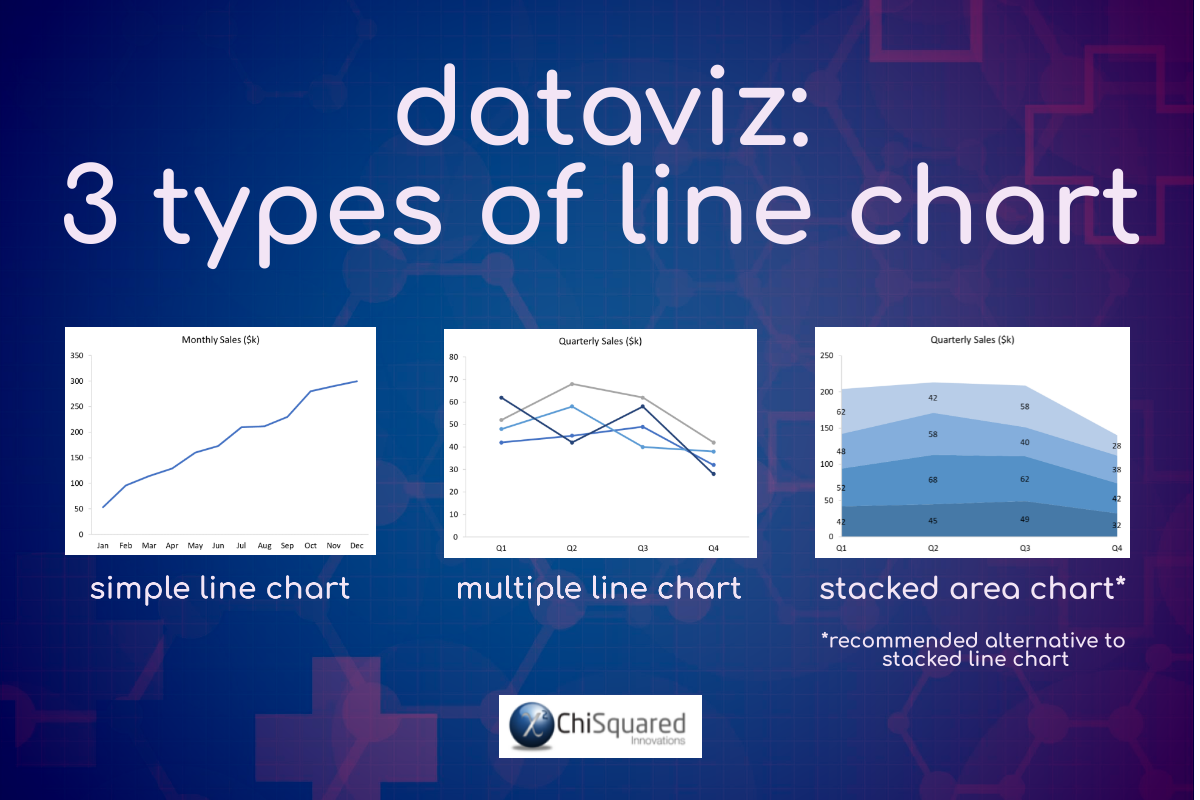If you would like to learn more about Line Charts, here is a much more in-depth article (opens in a new tab):

## Line Charts – What Are You Missing in Your Analysis?

### Scatter Plot

#### What does a Scatter Plot show?

A Scatter Plot shows the relationship between a pair of numerical variables. Before plotting your data, you should identify the variable with your feature of interest and plot this on the y-axis.

#### When To Use Scatter Plots

Scatter Plots are used when you’re looking for relationships between numerical variables, and are often accompanied by a trend line to help show correlations.

When the trend line rises from bottom left to top right, there is a positive correlation between the variables (this is not necessarily significant – you need a statistics test to confirm this), i.e. when the predictor variable (x-axis) increases, your feature of interest (y-axis) increases too.

When the trend line falls from top left to bottom right, there is a negative correlation – as your predictor variable increases, your feature of interest decreases.

When the trend line is (mostly) horizontal or vertical, there is no relationship between the variables.

Scatter Plots are particularly good for spotting outliers in your data set.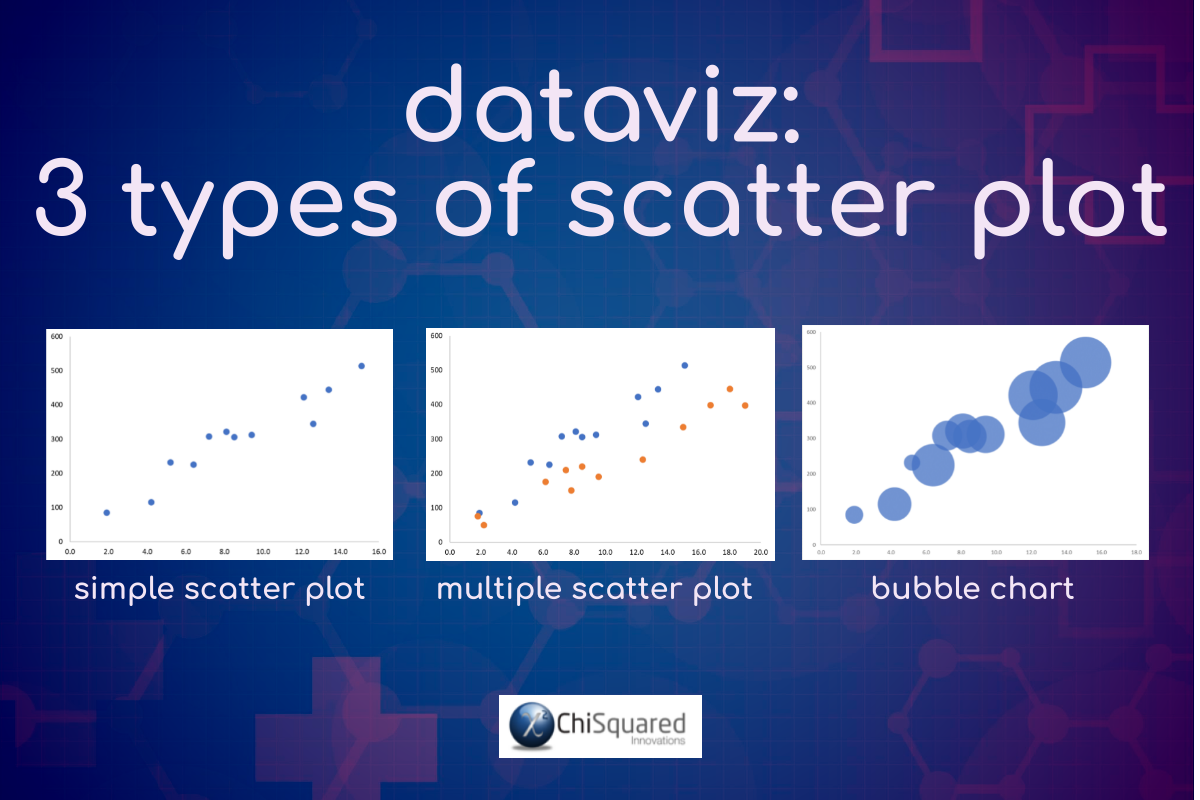If you would like to learn more about Scatter Plots, here is a much more in-depth article (opens in a new tab):

## Scatter Plots – What Separates the Pros from the Novices

### Bubble Chart

#### What does a Bubble Chart show?

A Bubble Chart is similar to a Scatter Plot but is used when there is a third numerical variable, which is shown as the size of the bubble, and is very powerful for visualising 2 or more variables with multiple dimensions.

#### When To Use Bubble Charts

You would use a Bubble Chart (3 numerical variables) when a Scatter Plot (2 numerical variables) is appropriate but you have an additional dimension that you want to show.

Bubble Charts are particularly useful when you wish to present patterns in large data sets, trends, correlations, clusters or outliers.